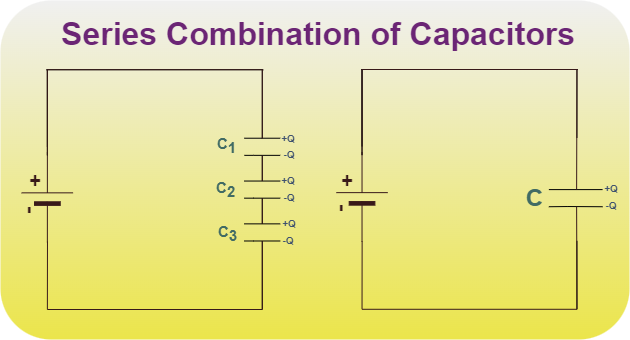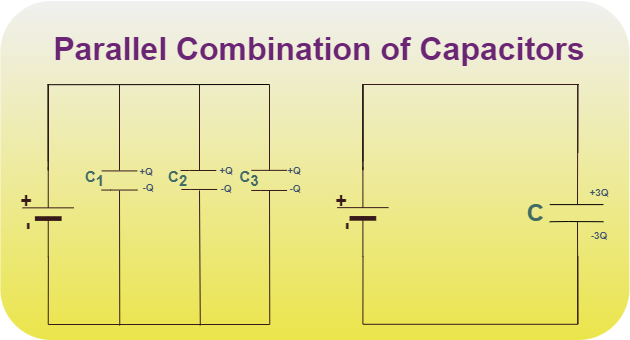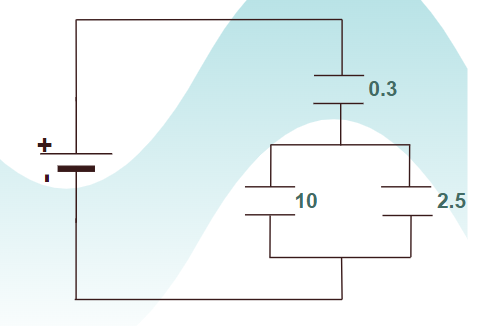Related Articles
• Explore Our Geeks Community
• CBSE Class 12 Physics Notes

# Capacitors in Series and Parallel

Capacitors are special devices that can hold electric charges for instantaneous release in an electric circuit. We can easily connect various capacitors together as we connected the resistor together. The capacitor can be connected in series or parallel combinations and can be connected as a mix of both.

In this article, we will learn about capacitors connected in series and parallel, their examples, and others in detail.

## Capacitor Definition

Capacitor is defined as follows:

Capacitors are electrical devices that store electrical energy in the circuit developed due to the opposite charges deposited on each plate due to the electrical field.

## Capacitance Definition

Capacitance is defined as the charge-storing capacity of an electrical device. It is given by C = q/V where C is capacitance, q is charge and V is voltage. The unit of Capacitance is Farad denoted by “F”.

## How are Capacitors Connected?

We can arrange for various capacitors to be connected with each other and the total capacitance of all the capacitors can be defined as the ratio of the total charge held by the capacitor and the total voltage applied in the circuit. This can be represented as,

Ceq = Q/V

where C is Capacitance

Q is Charge

V is Voltage

Now we can connect various capacitors in two configurations and the two configurations are,

• Series Combination
• Parallel Combination

## Series Combination of Capacitors

In the figure given below, three capacitors are connected in series with the battery of voltage V. Note that in the figure, opposite charges of equal magnitude flow and get accumulated on the plates of the capacitor. Conservation of charge principles requires that the charge that is accumulated on the plates of the capacitor must be equal in magnitude. The end result is a combination that resembles a single capacitor with an effective plate separation that is greater than that of individual capacitors, this equivalent capacitor is shown in the figure below. Large plate separation means smaller capacitance.The derivation of relation for capacitors in series is explained below:

The relation for capacitance is given by,

C = Q/V

It can be rewritten as,

V = Q/C

The voltages across individual capacitors will be,

V1 = Q/C1 , V2 = Q/C2, V3 = Q/C3

The total voltage across all the capacitors will be,

V = V1 + V2 + V3

substituting the expressions for individual voltages,

V = Q/C1 +Q/C + Q/C3

Let the equivalent capacitance be C,

Q/C = Q/C1 +Q/C + Q/C3

Upon Simplifying the above equation, the relation becomes,In general for capacitors C1, C2, C3,…### Example of Capacitor Connected in Series Combination

Let’s take four capacitors of capacitance 2 μF, 6 μF, 8 μF, and 3 μF connected in series then find the equivalent capacitance of the circuit.

Solution:

Given

• C1 = 2 μF
• C2 = 6 μF
• C3 = 8 μF
• C4 = 3 μF

Equivalent capacitance of the capacitor in Parallel Combination

1/Ceq = 1/C1 + 1/C2 + 1/C3 + 1/C4

1/Ceq = 1/2 + 1/6 + 1/8 + 1/3

1/Ceq = (12 + 4 + 3 + 8)/24 = 27/ 24

Ceq = 24/27 μF

Thus, the equivalent capacitance of the capacitor connected in series is, 24/27 μF

## Parallel Combination of Capacitors

In the figure given below, three capacitors C1, C2, and C3 are connected in parallel to a voltage source of potential V. Deriving the equivalent capacitance for this case is relatively simple. Note that the voltage across each capacitor is the same as that of the source since it is directly connected to the source. Thus capacitors have the same charges on them as they would have if connected individually to the voltage source.The derivation of the relation for Capacitors in Parallel is explained below:

Let’s say the total charge on the capacitors is Q,

Q = Q1 + Q2 + Q3

Q = Q1 + Q2 + Q3

CV = C1V + C2V + C3V

C = C1 + C2 + C3

### Example of Capacitor Connected in Parallel Combination

Let’s take four capacitors of capacitance 2 μF, 6 μF, 8 μF, and 3 μF connected in parallel then find the equivalent capacitance of the circuit.

Solution:

Given

• C1 = 2 μF
• C2 = 6 μF
• C3 = 8 μF
• C4 = 3 μF

Equivalent capacitance of the capacitor in Parallel Combination

Ceq = C1 + C2 + C3 + C4

Ceq = 2 + 6 + 8 + 3

Ceq = 19 μF

Thus, the equivalent capacitance of the capacitor connected in parallel is, 19 μF

### Some Important Points

• The capacitance of N identical capacitors connected in series combination,

1/Ceq = 1/C + 1/C + 1/C +…+ 1/C(n times)

1/Ceq = N/C

Ceq = C/N

• The capacitance of N identical capacitors connected in parallel combination,

Ceq = C + C + C +…+ C(n times)

Ceq = NC

## Examples on Combination of Capacitors

Example 1: Find the equivalent capacitance for the system of capacitors 3 pF, 5 pF, and 10 pF added in parallel combination.

Solution:

Formula for Combintion of Capcitor in Parallel Combination is,

C = C1 + C2 + C3

Given,

• C1 = 3 pF
• C = 5 pF
• C3 = 10 pF

Substituting these values we get,

C = 3 + 5 + 10

C = 18 pF

Thus, the equivalent capacitance of given capacitor is 18 pF

Example 2: Find the equivalent capacitance for the system of capacitors 2 pF, 2 pF, and 4 pF added in a series combination.

Solution:

Formula for Combintion of Capcitor in Series Combination is,Given,

• C1 = 2 pF
• C = 2 pF
• C3 = 4 pF

Substituting these values we get,C = 4/5

Thus, the equivalent capacitance of given capacitor is 4/5 pF.

Example 3: Find the equivalent capacitance for the system shown in the figure below.Solution:

Formula for Combintion of Capcitor in Parallel and Series Combination is,

•• C = C1 + C2 + C + ….

Given,

• C1 = 10 μF,
• C = 2.5 μF

C = C1 + C2

C = 10 + 2.5

C = 12.5C = 0.29

Thus, the equivalent capacitance of given system is 0.29 μF

Example 4: If the equivalent capacitance for the system of capacitors x, 5 pF, and 10 pF added in parallel is 23 pF. Find the value of x.

Solution:

Formula for Combintion of Capcitor in Parallel Combination is,

C = C1 + C2 + C3

Given,

• C1 = x
• C = 5 pF
• C3 = 10 pF

Substituting these values we get,

23 = x + 5 + 10

x = 23 – 15 = 8 pF

x = 8 pF

Thus, the required capacitance of given capacitor is 8 pF.

Example 5: If the equivalent capacitance for the system of capacitors 3 F,  6 F, and x F added in parallel is 6/7 F

Solution:

Formula for Combintion of Capcitor in Parallel Combination is,Given,

• C = 6/7 F
• C1 = 2 F
• C = 2 F
• C3 = x F

Substituting these values we get,7/6 = (2+1)/6 + 1/x

7/6 = 3/6 + 1/x

7/6 – 3/6 = 1/x

4/6 = 1/x

2/3 = 1/x

x = 3/2

Thus, the value of unknown resitance x is 3/2 F.

## FAQs on Capacitance

### Q1: What is Capacitor?

Capacitors are electrical instruments that are used to store energy in the form of charge in an electric circuit.

### Q2: What is Capacitance?

Capacitance is the property of any material to store charges. And the material that stores charges are called a capacitor.

### Q3: How to store Energy in Capacitor?

The energy in the capacitor is stored as Electrical Potential Energy. The change in the voltage of the capacitor results in a change in its potential energy.